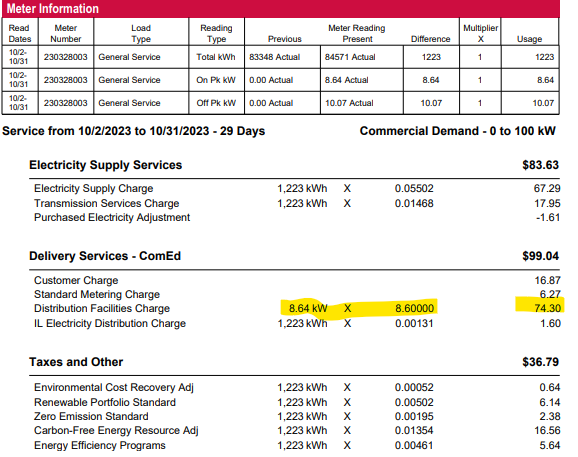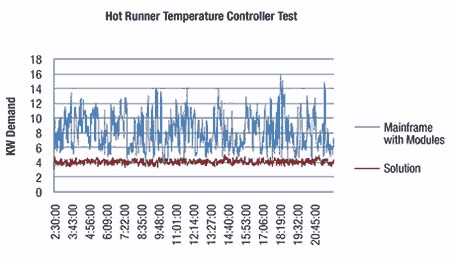An electricity bill has two main usage categories: kilowatt-hours (kWh) and kW demand. kWh is a calculation of wattage multiplied by the number of hours used, divided by 1000. We pay approximately \$.10/kWh used in a given month. kW demand is the highest kW recorded during peak hours and may bill around \$9.00 per kW. How high of a kW demand does the electric company have allocated to you? The higher you peak, the more it costs.

A given injection mold and hot runner system will require a certain amount of wattage to achieve and maintain the setpoint. That is just a factor of the tool’s heat/loss design. Once the tool is designed and built, “it is what it is,” as they say. The wattage required is the wattage required, albeit some processing tweaks may allow for lower operating temperatures, and less “over-under” shooting by your hot runner controller can reduce waste. The most crucial factor is that we can control when the wattage is applied, which can drastically reduce the kW demand portion of an electric bill. So, let’s look at an example tool:

• 72 zones total
• 8 manifold zones at 20 amps each (4800-watt heaters)
• 64 nozzle zones at 3.12 amps each (748.8-watt heaters)
• 359.7 total system amps
• Ramp-up time at 100% power (how long each zone takes to go from ambient temperature to the set point). For this example, manifold zones take 10 minutes, and the nozzles 5 minutes.
• 8 zones x 4800 watts/ea x 100% power divided by 1,000 watts/kW x 10 min (.17 hrs)  = 6.53 kWh with a peak demand of 38.4 kW (8 x 4.8)
• 64 zones x 748.8 watts/ea x 100% power divided by 1,000 watts/kW x 5 min (.085 hrs) = 4.73 kWh with peak demand of 47.9 kW (64 x .7488)
• Setpoint operation per hour at an estimated 10% power
• 8 zones x 4800 watts/ea x 10% power divided by 1,000 watts/kW x 1 hr  = 3.84 kWh with a peak demand of 38.4 kW (8 x 4.8)
• 64 zones x 748.8 watts/ea x 10% power divided by 1,000 watts/kW x 1 hr = 4.79 kWh with peak demand of 47.9 kW (64 x .7488)
• kW Demand is now the total of manifold zones and nozzles zones because they are operating at the same time. 38.4 kW + 47.9 kW = 86.33 kW

Using our \$.10/kWh, here is the kWh cost per start-up (ramp-up) and per hour of setpoint operation:

• Start-up (6.53 kWh + 4.73 kWh) x \$.10/kWh = \$1.13
• Hourly operation at setpoint (3.84 kWh + 4.79 kWh) x \$.10/kWh = \$.86/hr
• A 2 shift/day x 5 days per week scenario:
• 1 start-up and approximately 16 hrs of setpoint operation per day would be \$1.13 + (16 x \$.86) = \$13.29
• 4 weeks/mo would be \$13.29 x 4 = \$53.16/mo

Compare that to the higher kW demand recorded for the total system 86.33 kW x \$9/kW = \$776.70! Reducing kW demand is the most significant opportunity for saving on electrical costs. Of course, this is just one tool in a molding facility of many tools and various equipment, so you would have to compare the peak kW Demand of those other sources to see what drives a particular facility’s electric bill.

## How to Reduce kW Demand (Peak Amp Draw) of a Hot Runner System

The total system amps for the example tool above are 359.7 (86.33 kW) if all zones can turn on simultaneously. There are two reasons you would want to reduce the kW demand, also known as peak amp draw. The first is the potential electrical savings, as outlined above. The second reason to reduce the peak amp draw is to help balance the electrical load within a facility and enable the hot runner system to operate on as small of an amp supply as possible.

There are three ways that our Pulse hot runner controller reduces kW demand.

1. Waterfall Technology – Pulse automatically splits the total zones into three groups, one for each phase. Each phase is allowed to turn on in a staggered sequence. This layered approach can reduce peak amp draw by up to 67% when maintaining zone setpoint for most applications.
2. EvenSoak – You can break the hot runner system into ramp-up groups (which you should do for proper steel expansion anyway). Examples of groups would be primary manifolds, secondary manifolds, sprue, and sections of nozzles. This way, you only apply 100% power to one group at a time.  [What does 100% power mean? It means that for a set group of alternating current half-cycles within a control loop, 100% of those half-cycles will be on.]
3. Max % Power – The Pulse hot runner controller allows you to set the % power to less than 100%. For example, if % power is 50%, then within the control loop, the first 50% of the half cycles would be on, and the second 50% would be off. The combination of Max % Power and Waterfall Technology™ means you could reduce peak amp draw by up to 67% when max % power is 33%. If you set the max % power at 67%, you would reduce the peak amp draw by 33%.

Utilizing these tools would reduce the 86.33 kW demand for our example tool by 67%!

We have performed several live tests over the years. The example below is a test performed with Florida Power and Light and a customer’s facility. It shows that kW demand using Pulse is 1/3 of a traditional mainframe controller.